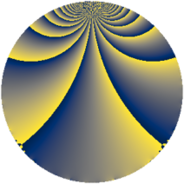# Properties

 Label 380.2.nLevel $380$ Weight $2$ Character orbit 380.n Rep. character $\chi_{380}(31,\cdot)$ Character field $\Q(\zeta_{6})$ Dimension $80$ Newform subspaces $2$ Sturm bound $120$ Trace bound $2$

# Related objects

## Defining parameters

 Level: $$N$$ $$=$$ $$380 = 2^{2} \cdot 5 \cdot 19$$ Weight: $$k$$ $$=$$ $$2$$ Character orbit: $$[\chi]$$ $$=$$ 380.n (of order $$6$$ and degree $$2$$) Character conductor: $$\operatorname{cond}(\chi)$$ $$=$$ $$76$$ Character field: $$\Q(\zeta_{6})$$ Newform subspaces: $$2$$ Sturm bound: $$120$$ Trace bound: $$2$$ Distinguishing $$T_p$$: $$3$$

## Dimensions

The following table gives the dimensions of various subspaces of $$M_{2}(380, [\chi])$$.

Total New Old
Modular forms 128 80 48
Cusp forms 112 80 32
Eisenstein series 16 0 16

## Trace form

 $$80 q + 2 q^{4} - 2 q^{6} - 44 q^{9} + O(q^{10})$$ $$80 q + 2 q^{4} - 2 q^{6} - 44 q^{9} - 6 q^{10} + 24 q^{13} + 2 q^{16} - 8 q^{17} + 24 q^{21} + 12 q^{24} - 40 q^{25} + 36 q^{26} + 20 q^{28} + 16 q^{30} - 30 q^{32} - 12 q^{33} - 54 q^{34} - 20 q^{36} + 50 q^{38} + 72 q^{41} - 42 q^{42} + 20 q^{44} + 18 q^{48} - 120 q^{49} - 18 q^{52} - 24 q^{53} - 22 q^{54} + 40 q^{57} - 60 q^{58} + 6 q^{60} + 16 q^{61} - 80 q^{62} - 16 q^{64} + 20 q^{66} + 52 q^{68} + 36 q^{70} + 84 q^{72} - 36 q^{73} + 12 q^{74} - 32 q^{76} - 16 q^{77} + 120 q^{78} + 16 q^{80} - 32 q^{81} - 42 q^{82} - 16 q^{85} + 96 q^{86} - 36 q^{89} + 78 q^{90} + 56 q^{92} - 8 q^{93} - 152 q^{96} + 12 q^{97} - 78 q^{98} + O(q^{100})$$

## Decomposition of $$S_{2}^{\mathrm{new}}(380, [\chi])$$ into newform subspaces

Label Dim $A$ Field CM Traces $q$-expansion
$a_{2}$ $a_{3}$ $a_{5}$ $a_{7}$
380.2.n.a $40$ $3.034$ None $$-3$$ $$0$$ $$20$$ $$0$$
380.2.n.b $40$ $3.034$ None $$3$$ $$0$$ $$-20$$ $$0$$

## Decomposition of $$S_{2}^{\mathrm{old}}(380, [\chi])$$ into lower level spaces

$$S_{2}^{\mathrm{old}}(380, [\chi]) \cong$$ $$S_{2}^{\mathrm{new}}(76, [\chi])$$$$^{\oplus 2}$$